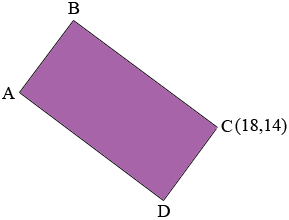SEARCH HOMEMath Central Quandaries & QueriesQuestion from Mitchell, a student: The perimeter of rectangle ABCD (represented on a Cartesian plane) is 52cm. The equation associated with segment AD is 3x+4y-60=0 Point C is at (18, 14). What is the length of segment AD?Mitchell,

I redrew the diagram that you sent.Write the equation of the line through AD, 3x + 4y - 60 = 0, in the form y = mx + b. I got

y = -3/4 x + 15/2.               (*)

Thus the slope of this line is -3/4. Since ABCD is a rectangle CD is perpendicular to AD and thus its slope is the negative reciprocal of -3/4, that is 4/3.

Write the equation of the line through (18, 14) with slope 4/3. Solve this equation with equation (*) to find the coordinates of D. Find the distance between C and D. Since you know the perimeter is 52 cm you can now find the length of the segment AD.

PennyMath Central is supported by the University of Regina and The Pacific Institute for the Mathematical Sciences.